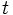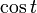# Cosine function

Jump to: navigation, search
This article is about a particular function from a subset of the real numbers to the real numbers. Information about the function, including its domain, range, and key data relating to graphing, differentiation, and integration, is presented in the article.
View a complete list of particular functions on this wiki
For functions involving angles (trigonometric functions, inverse trigonometric functions, etc.) we follow the convention that all angles are measured in radians. Thus, for instance, the angle of$90\,^\circ$ is measured as$\pi/2$.

## Definition

### Unit circle definition

The cosine function, denoted$\cos$, is defined as follows.

Consider the unit circle centered at the origin, described as the following subset of the coordinate:$\{ (x,y) \mid x^2 + y^2 = 1\}$

For a real number$t$, we define$\cos t$ as follows:

• Start at the point$(1,0)$, which lies on the unit circle centered at the origin.
• Move a distance of$t$ along the unit circle in the counter-clockwise direction (i.e., the motion begins in the first quadrant, with both coordinates positive).
• At the end, the$x$-coordinate of the point thus obtained is defined as$\cos t$.

### Triangle ratio definition (works for acute angles)

For an acute angle$t$, i.e., for$t$ in the open interval$(0,\pi/2)$,$\cos t$ can be defined as follows:

• Construct any right triangle with one of the acute angles equal to$t$.
•$\! \cos t$ is the ratio of the leg adjacent to the angle$t$ to the hypotenuse.